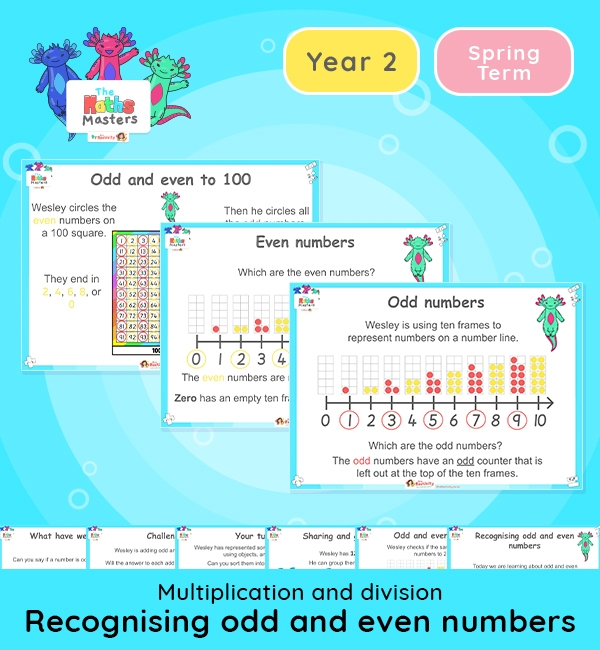# Year 2 | Recognising Odd and Even Numbers Lesson Presentation## White Rose Year 2 multiplication and division lesson plans

Aligned with the White Rose Maths scheme of work, this Year 2 | Recognising Odd and Even Numbers Lesson Presentation is fully editable, and is designed for the Year 2 maths curriculum covering the following maths objectives for the autumn term:

Topic: Multiplication and division

This lesson focuses on recognising and exploring odd and even numbers.

White Rose Maths Small Steps: Odd and even numbers.

NC Links: Recall and use multiplication and division facts for the 2, 5, and 10 times tables including recognising odd and even numbers. *Solve problems involving multiplication and division using materials, arrays, repeated addition, mental methods and multiplication and division facts including problems in context. *Count in steps of 2, 3, 5, and 10.

TAF Statements: Working At  – Recall and use multiplication and division facts for 2, 5, and 10 and use them to solve simple problems.

Greater Depth – Use reasoning about number and relationships to solve more complex problems and explain their thinking.

Ready-to-progress criteria:  2MD-1 Recognise repeated addition contexts, representing them with multiplication equations and calculating the product within the 2, 5, and 10 multiplication tables.

Year 1 conceptual prerequisites: Count in multiples of 2, 5 and 10

Future applications: Use multiplication to represent repeated addition contexts for other group sizes

## Recently Viewed